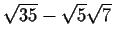Next: Algebraic numbers Up: Modular mappings Previous: Complex numbers

## Square roots of integers

To find the signatures of square roots of integers (everything else is recursively computed to an integer) we need to resolve two problems.

(a) Some integers do not have a square root mod n.

(b) When we find a square root, we have two choices (sign choice). We must have a consistent way of choosing the sign so that we will not make mistakes with expressions like. When n is not prime there will be more than two choices for the square root.

Both of these problems are also problems with algebraic numbers and will be discussed in the next part. If we are using a field extension for the representation of i, then all square roots can be represented, either by an integer or by a multiple of the field extension.

Gaston Gonnet
1999-07-04